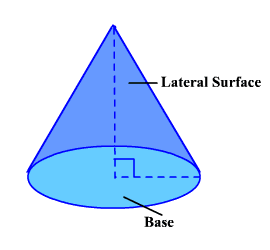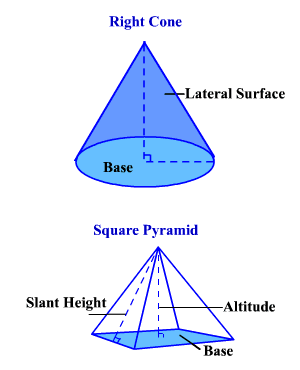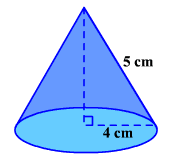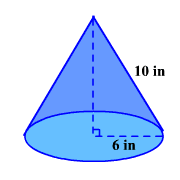# Surface Area of a Cone

The total surface area of a cone is the sum of the area of its base and the lateral (side) surface.

The lateral surface area of a cone is the area of the lateral or side surface only.Since a cone is closely related to a pyramid , the formulas for their surface areas are related.Remember, the formulas for the lateral surface area of a pyramid is $\frac{1}{2}pl$ and the total surface area is $\frac{1}{2}pl+B$ .

Since the base of a cone is a circle, we substitute $2\pi r$ for $p$ and $\pi {r}^{2}$ for $B$ where $r$ is the radius of the base of the cylinder.

So, the formula for the lateral surface area of a right cone is $L.S.A=\pi rl$ , where $l$ is the slant height of the cone .

Example 1:

Find the lateral surface area of a right cone if the radius is $4$ cm and the slant height is $5$ cm.$L.S.A=\pi \left(4\right)\left(5\right)=20\pi \approx 62.82\text{\hspace{0.17em}}\text{\hspace{0.17em}}{\text{cm}}^{2}$

The formula for the total surface area of a right cone is $T.S.A=\pi rl+\pi {r}^{2}$ .

Example 2:

Find the total surface area of a right cone if the radius is $6$ inches and the slant height is $10$ inches.$\begin{array}{l}T.S.A=\pi \left(6\right)\left(10\right)+\pi {\left(6\right)}^{2}\\ =60\pi +36\pi \\ =96\pi \text{\hspace{0.17em}}{\text{inches}}^{2}\\ \approx 301.59\text{\hspace{0.17em}}{\text{inches}}^{2}\end{array}$